# KSEEB SSLC Class 10 Maths Solutions Chapter 8 Real Numbers Ex 8.4

KSEEB SSLC Class 10 Maths Solutions Chapter 8 Real Numbers Ex 8.4 are part of KSEEB SSLC Class 10 Maths Solutions. Here we have given Karnataka SSLC Class 10 Maths Solutions Chapter 8 Real Numbers Exercise 8.4.

## Karnataka SSLC Class 10 Maths Solutions Chapter 8 Real Numbers Exercise 8.4

Question 1.
Without actually performing the long division, state whether the following rational numbers will have a terminating decimal expansion or a non-terminating repeating decimal expansion :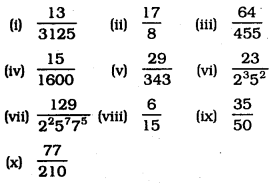Solution:
(i)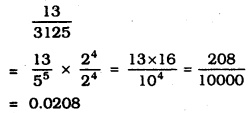∴ This is terminating decimal expansion.

(ii)∴ This is terminating decimal expansion.

(iii)
$$\frac{64}{455}=\frac{2^{6}}{5 \times 7 \times 13}$$
∴ This is non-terminating repeating expansion.

(iv)∴ This is terminating decimal expansion.

(v)
$$\frac{29}{343}=\frac{29}{7^{3}}$$
∴ This is non-terminating repeating expansion.

(vi)∴ This is terminating decimal expansion.

(vii)
$$\frac{129}{2^{2} 5^{7} 7^{5}}$$
∴ This is non-terminating repeating expansion.

(viii)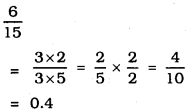∴ This is terminating decimal expansion.

(ix)∴ This is terminating decimal expansion.

(x)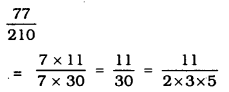∴ This is non-terminating repeating expansion.

Question 2.
Write down the decimal expansions of those rational numbers in Question 1 above which have terminating decimal expansions.
Solution: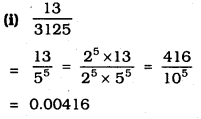Question 3.
The following real numbers have decimal expansions as given below. In each case, decide whether they are rational or not. If they are rational, and of the form $$\frac{p}{q}$$, what can you say about the prime factors of q?
(i) 43.123456789
(ii) 0.120120012000120000………..
(iii) $$43 . \overline{123456789}$$
Solution:
(i) 43.123456789
$$\frac{p}{q}$$ is a rational number
Prime factor of q:
q = 109 = 29 × 59

(ii) 0.120120012000120000…………
This is an irrational number

(iii) $$43 . \overline{123456789}$$
This is a rational number.
Because, Prime factors of ‘q’ are contrinued in non-terminating decimal expansion.

We hope the given KSEEB SSLC Class 10 Maths Solutions Chapter 8 Real Numbers Ex 8.4 will help you. If you have any query regarding Karnataka SSLC Class 10 Maths Solutions Chapter 8 Real Numbers Exercise 8.4, drop a comment below and we will get back to you at the earliest.

error: Content is protected !!